# Open: EfficiencyOfExchangeabilityMartingales

There are two different versions of this question:

According to de Finetti's theorem, the difference between the assumptions of randomness and exchangeability disappears (under very weak assumptions about the observation space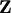) for infinite sequences of observations, since any exchangeable probability distribution is a mixture of IID distributions. For finite sequences of observations, however, there is a difference: e.g., in the case of binary sequences,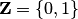, it was shown by Vovk (1986), using the language of Kolmogorov's theory of randomness, that a finite binary sequence is random with respect to the IID model if and only if it is exchangeable and the number of 1s in it is random with respect to the binomial model.

One specific version of the question is about testing the assumption of exchangeability for finite sequences of a given length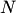. In the simplest version the sequences are binary. Consider a set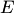in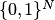whose probability is at most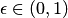under any exchangeable probability distribution on. Does there exist a nonnegative conformal martingale that starts from(or a number not much greater thanfor a small) and ends up with at least 1 on any sequence in. (Cf. the definition of game-theoretic probability.) Since conformal martingales are randomized, the condition that the final value is at least 1 can be formalized in different way. The strongest formalization is that it should hold almost surely (over the internal coin tosses of the conformal martingale). A weak formalization is to require that the condition holds on average over the internal coin tosses.

### First step towards a solution

Let us consider the following singleton set: its only element is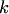1s followed by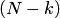0s. (This is a toy version of the problem of anomaly detection.) The supremum of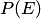over all exchangeable probability distributions is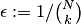. There is a deterministic nonnegative conformal martingale that starts fromand ends up with 1 on the event. Such a conformal martingale can be obtained from the conformity measure that always regards 0 as less typical than 1 and the base martingale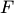that starts fromand satisfies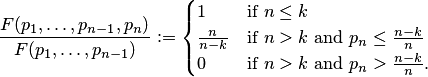It is easy to check that all these statements remain true for any singleton, withbeing the number of 1s in the only element of.

Bibliography